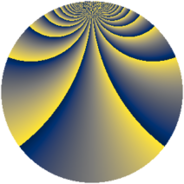# Properties

 Label 2004.2.iLevel $2004$ Weight $2$ Character orbit 2004.i Rep. character $\chi_{2004}(25,\cdot)$ Character field $\Q(\zeta_{83})$ Dimension $2296$ Sturm bound $672$

# Related objects

## Defining parameters

 Level: $$N$$ $$=$$ $$2004 = 2^{2} \cdot 3 \cdot 167$$ Weight: $$k$$ $$=$$ $$2$$ Character orbit: $$[\chi]$$ $$=$$ 2004.i (of order $$83$$ and degree $$82$$) Character conductor: $$\operatorname{cond}(\chi)$$ $$=$$ $$167$$ Character field: $$\Q(\zeta_{83})$$ Sturm bound: $$672$$

## Dimensions

The following table gives the dimensions of various subspaces of $$M_{2}(2004, [\chi])$$.

Total New Old
Modular forms 28044 2296 25748
Cusp forms 27060 2296 24764
Eisenstein series 984 0 984

## Trace form

 $$2296q - 28q^{9} + O(q^{10})$$ $$2296q - 28q^{9} + 4q^{11} - 4q^{13} - 4q^{15} - 16q^{23} - 40q^{25} - 4q^{29} - 8q^{33} + 4q^{35} - 16q^{37} + 8q^{39} - 4q^{41} - 4q^{47} - 20q^{49} - 8q^{53} + 8q^{55} - 8q^{57} + 12q^{59} - 16q^{61} - 36q^{65} - 24q^{67} + 4q^{69} + 12q^{71} + 24q^{73} - 8q^{75} - 16q^{77} + 12q^{79} - 28q^{81} + 8q^{83} - 36q^{85} - 20q^{89} + 16q^{91} - 52q^{97} + 4q^{99} + O(q^{100})$$

## Decomposition of $$S_{2}^{\mathrm{new}}(2004, [\chi])$$ into newform subspaces

The newforms in this space have not yet been added to the LMFDB.

## Decomposition of $$S_{2}^{\mathrm{old}}(2004, [\chi])$$ into lower level spaces

$$S_{2}^{\mathrm{old}}(2004, [\chi]) \cong$$ $$S_{2}^{\mathrm{new}}(167, [\chi])$$$$^{\oplus 6}$$$$\oplus$$$$S_{2}^{\mathrm{new}}(334, [\chi])$$$$^{\oplus 4}$$$$\oplus$$$$S_{2}^{\mathrm{new}}(501, [\chi])$$$$^{\oplus 3}$$$$\oplus$$$$S_{2}^{\mathrm{new}}(668, [\chi])$$$$^{\oplus 2}$$$$\oplus$$$$S_{2}^{\mathrm{new}}(1002, [\chi])$$$$^{\oplus 2}$$